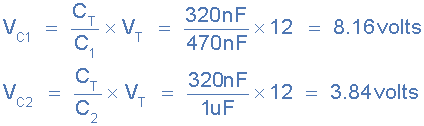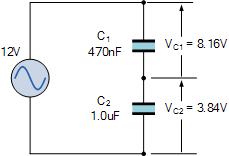Capacitors are connected together in series when they are daisy chained together in a single line.

For series connected capacitors, the charging current ( iC ) flowing through the capacitors is THE SAME for all capacitors as it only has one path to follow.

Then, Capacitors in Series all have the same current flowing through them as iT = i1 = i2 = i3 etc. Therefore each capacitor will store the same amount of electrical charge, Q on its plates regardless of its capacitance. This is because the charge stored by a plate of any one capacitor must have come from the plate of its adjacent capacitor. Therefore, capacitors connected together in series must have the same charge.

QT = Q1 = Q2 = Q3 ….etc

Consider the following circuit in which the three capacitors, C1C2 and C3  are all connected together in a series branch across a supply voltage between points A and B.

### Capacitors in a Series ConnectionIn the previous parallel circuit we saw that the total capacitance, CT of the circuit was equal to the sum of all the individual capacitors added together. In a series connected circuit however, the total or equivalent capacitance CTis calculated differently.

In the series circuit above the right hand plate of the first capacitor, C1 is connected to the left hand plate of the second capacitor, C2 whose right hand plate is connected to the left hand plate of the third capacitor, C3. Then this series connection means that in a DC connected circuit, capacitor C2 is effectively isolated from the circuit.

The result of this is that the effective plate area has decreased to the smallest individual capacitance connected in the series chain. Therefore the voltage drop across each capacitor will be different depending upon the values of the individual capacitance’s.

Then by applying Kirchhoff’s Voltage Law, ( KVL ) to the above circuit, we get:Since Q = C*V and rearranging for V = Q/C, substituting Q/C for each capacitor voltage VC in the above KVL equation will give us:dividing each term through by Q gives

### Series Capacitors EquationWhen adding together Capacitors in Series, the reciprocal ( 1/C ) of the individual capacitors are all added together ( just like resistors in parallel ) instead of the capacitance’s themselves. Then the total value for capacitors in series equals the reciprocal of the sum of the reciprocals of the individual capacitances.

## Capacitors in Series Example No1

Taking the three capacitor values from the above example, we can calculate the total capacitance, CT for the three capacitors in series as:One important point to remember about capacitors that are connected together in a series configuration, is that the total circuit capacitance ( CT ) of any number of capacitors connected together in series will always be LESS than the value of the smallest capacitor in the series and in our example above CT = 0.055μF with the value of the smallest capacitor in the series chain is only 0.1μF.

This reciprocal method of calculation can be used for calculating any number of individual capacitors connected together in a single series network. If however, there are only two capacitors in series, then a much simpler and quicker formula can be used and is given as:If the two series connected capacitors are equal and of the same value, that is: C1 = C2, we can simplify the above equation further as follows to find the total capacitance of the series combination.Then we can see that if and only if the two series connected capacitors are the same and equal, then the total capacitance, CT will be exactly equal to one half of the capacitance value, that is: C/2.

With series connected resistors, the sum of all the voltage drops across the series circuit will be equal to the applied voltage VS ( Kirchhoff’s Voltage Law ) and this is also true about capacitors in series.

With series connected capacitors, the capacitive reactance of the capacitor acts as an impedance due to the frequency of the supply. This capacitive reactance produces a voltage drop across each capacitor, therefore the series connected capacitors act as a capacitive voltage divider network.

The result is that the voltage divider formula applied to resistors can also be used to find the individual voltages for two capacitors in series. Then:Where: CX is the capacitance of the capacitor in question, VS is the supply voltage across the series chain and VCX is the voltage drop across the target capacitor.

## Capacitors in Series Example No2

Find the overall capacitance and the individual rms voltage drops across the following sets of two capacitors in series when connected to a 12V AC supply.

• a)  two capacitors each with a capacitance of 47nF
• b)  one capacitor of 470nF connected in series to a capacitor of 1μF

a) Total Equal Capacitance,Voltage drop across the two identical 47nF capacitors,b) Total Unequal Capacitance,Voltage drop across the two non-identical Capacitors: C1 = 470nF and C2 = 1μF.Since Kirchhoff’s voltage law applies to this and every series connected circuit, the total sum of the individual voltage drops will be equal in value to the supply voltage, VS. Then 8.16 + 3.84 = 12V.

Note also that if the capacitor values are the same, 47nF in our first example, the supply voltage will be divided equally across each capacitor as shown. This is because each capacitor in the series chain shares an equal and exact amount of charge ( Q = C x V = 0.564μC ) and therefore has half ( or percentage fraction for more than two capacitors ) of the applied voltage, VS.

However, when the series capacitor values are different, the larger value capacitor will charge itself to a lower voltage and the smaller value capacitor to a higher voltage, and in our second example above this was shown to be 3.84 and 8.16 volts respectively. This difference in voltage allows the capacitors to maintain the same amount of charge, Q on the plates of each capacitors as shown.Note that the ratios of the voltage drops across the two capacitors connected in series will always remain the same regardless of the supply frequency as their reactance, XC will remain proportionally the same.

Then the two voltage drops of 8.16 volts and 3.84 volts above in our simple example will remain the same even if the supply frequency is increased from 100Hz to 100kHz.

Although the voltage drops across each capacitor will be different for different values of capacitance, the coulomb charge across the plates will be equal because the same amount of current flow exists throughout a series circuit as all the capacitors are being supplied with the same number or quantity of electrons.

In other words, if the charge across each capacitors plates is the same, as Qis constant, then as its capacitance decreases the voltage drop across the capacitors plates increases, because the charge is large with respect to the capacitance. Likewise, a larger capacitance will result in a smaller voltage drop across its plates because the charge is small with respect to the capacitance.

## Capacitors in Series Summary

Then to summarise, the total or equivalent capacitance, CT of a circuit containing Capacitors in Series is the reciprocal of the sum of the reciprocals of all of the individual capacitance’s added together.

Also for capacitors connected in series, all the series connected capacitors will have the same charging current flowing through them as iT = i1 = i2 = i3etc. Two or more capacitors in series will always have equal amounts of coulomb charge across their plates.

As the charge, ( Q ) is equal and constant, the voltage drop across the capacitor is determined by the value of the capacitor only as V = Q ÷ C. A small capacitance value will result in a larger voltage while a large value of capacitance will result in a smaller voltage drop.